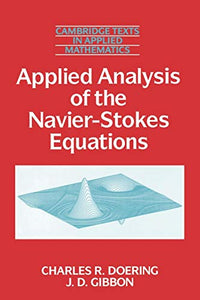# Applied Analysis of the Navier-Stokes Equations

Regular price
\$57.99
Sale price
\$57.99
Regular price
\$57.99
Sold out
Unit price
per
Shipping calculated at checkout.

 Author/Contributor(s): Doering, Charles R ; Gibbon, J D ; Doering Publisher: Cambridge University Press Date: 06/29/1995 Binding: Paperback Condition: NEW
The Navier-Stokes equations are a set of nonlinear partial differential equations that describe the fundamental dynamics of fluid motion. They are applied routinely to problems in engineering, geophysics, astrophysics, and atmospheric science. This book is an introductory physical and mathematical presentation of the Navier-Stokes equations, focusing on unresolved questions of the regularity of solutions in three spatial dimensions, and the relation of these issues to the physical phenomenon of turbulent fluid motion. The goal of the book is to present a mathematically rigorous investigation of the Navier-Stokes equations that is accessible to a broader audience than just the subfields of mathematics to which it has traditionally been restricted. Therefore, results and techniques from nonlinear functional analysis are introduced as needed with an eye toward communicating the essential ideas behind the rigorous analyses. This book is appropriate for graduate students in many areas of mathematics, physics, and engineering.